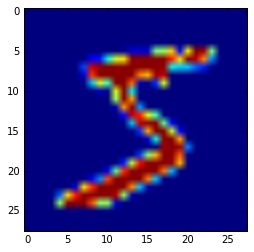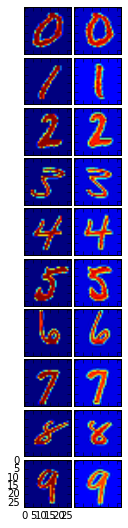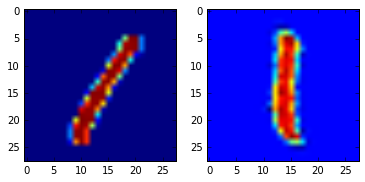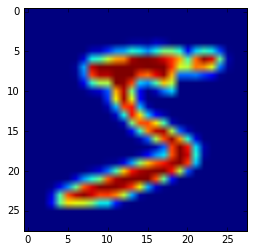In :
## Default imports: don't touch these
import numpy as np
import matplotlib.pyplot as plt
import scipy as sp
import math

In :
# More Boilerplate, don't worry
%matplotlib inline
from mnist import MNIST
mndata = MNIST('./%s/'%s)
X_train = X_train/255.0
X_test = X_test/255.0
return (X_train, labels_train), (X_test, labels_test)

In :
# Loaded the Libraries
(X_train, labels_train), (X_test, labels_test) = load_dataset()


# Deskewing¶

When we write, we often write at angles to the paper, which cause letters and numbers to be skewed. Unfortunately, unlike the human eye, computers cannot easily find similarities between images that are transformations of each other. Thus, the process of deskewing

Very formally, deskewing is the process of straightening an image that has been scanned or written crookedly — that is an image that is slanting too far in one direction, or one that is misaligned.

In particular, we model the process of deskewing as an affine transformation. We assume that when the image was created (the skewed version), it is actually some affine skew transformation on the image $Image' = A(Image) + b$ which we do not know. What we do know is that we want the center of mass to be the center of the image, and that we'd like to know the angle at which it was skewed.The methodology goes as follows:

1) Find the center of mass of the image to figure out how much we need to offset the image
2) Find the covariance matrix of the image pixel intensities (we can use this to approximate the skew of the angle)

The function moments below, calculates these relevant quantities

Next, we'd like to calculate the matrix which will allow us to skew "back" to the original image

This is given by the following formula

$$\begin{bmatrix}1 & 0 \\ \alpha & 1\end{bmatrix}$$

where $\alpha = \frac{Cov(X,Y)}{Var(X)}$

Furthermore, we have an offset of $\mu -$ center

Thus, combining the two, using the handy interpolation library from scipy, we complete the method deskew below.

Check out the results below!

In :
plt.imshow(X_train.reshape(28,28)) # This is what the image looks like

Out:
<matplotlib.image.AxesImage at 0x7fd3638ac828>In :
from scipy.ndimage import interpolation

def moments(image):
c0,c1 = np.mgrid[:image.shape,:image.shape] # A trick in numPy to create a mesh grid
totalImage = np.sum(image) #sum of pixels
m0 = np.sum(c0*image)/totalImage #mu_x
m1 = np.sum(c1*image)/totalImage #mu_y
m00 = np.sum((c0-m0)**2*image)/totalImage #var(x)
m11 = np.sum((c1-m1)**2*image)/totalImage #var(y)
m01 = np.sum((c0-m0)*(c1-m1)*image)/totalImage #covariance(x,y)
mu_vector = np.array([m0,m1]) # Notice that these are \mu_x, \mu_y respectively
covariance_matrix = np.array([[m00,m01],[m01,m11]]) # Do you see a similarity between the covariance matrix
return mu_vector, covariance_matrix

In :
def deskew(image):
c,v = moments(image)
alpha = v[0,1]/v[0,0]
affine = np.array([[1,0],[alpha,1]])
ocenter = np.array(image.shape)/2.0
offset = c-np.dot(affine,ocenter)
return interpolation.affine_transform(image,affine,offset=offset)

In :
examples = (4181, 0), (3,1), (56282, 2), (25829,3), (9741,4) , (26901,5), (50027,6), (17935,7) , (41495, 8), (14662, 9)

In :
from mpl_toolkits.axes_grid1 import AxesGrid
grid = AxesGrid(plt.figure(figsize=(8,15)), 141,  # similar to subplot(141)
nrows_ncols=(10, 2),
label_mode="1",
)

for examplenum,num in examples:
im = grid[2*num].imshow(X_train[examplenum].reshape(28,28))
im2 = grid[2*num+1].imshow(deskew(X_train[examplenum].reshape(28,28)))In :
plt.subplot(1, 2, 1)
plt.imshow(X_train.reshape(28,28))

newim = deskew(X_train.reshape(28,28))
plt.subplot(1, 2, 2)
plt.imshow(newim)

[[ 31.80782549 -17.91061544]
[-17.91061544  11.38495874]]

Out:
<matplotlib.image.AxesImage at 0x7fd367a2ec50>In :
import numpy as np
from scipy.ndimage.interpolation import map_coordinates
from scipy.ndimage.filters import gaussian_filter

def elastic_transform(image, alpha, sigma, random_state=None):
"""Elastic deformation of images as described in [Simard2003]_.
.. [Simard2003] Simard, Steinkraus and Platt, "Best Practices for
Convolutional Neural Networks applied to Visual Document Analysis", in
Proc. of the International Conference on Document Analysis and
Recognition, 2003.
"""
assert len(image.shape)==2

if random_state is None:
random_state = np.random.RandomState(None)

shape = image.shape

dx = gaussian_filter((random_state.rand(*shape) * 2 - 1), sigma, mode="constant", cval=0) * alpha
dy = gaussian_filter((random_state.rand(*shape) * 2 - 1), sigma, mode="constant", cval=0) * alpha

x, y = np.meshgrid(np.arange(shape), np.arange(shape), indexing='ij')
indices = np.reshape(x+dx, (-1, 1)), np.reshape(y+dy, (-1, 1))

return map_coordinates(image, indices, order=1).reshape(shape)

In :
newim2 = elastic_transform(X_train.reshape(28,28),36,6)
plt.imshow(newim2)

Out:
<matplotlib.image.AxesImage at 0x7f056a644c88>## Results¶

In :
from sklearn.preprocessing import OneHotEncoder
from sklearn import linear_model
import sklearn.metrics as metrics

In :
def createModel(x,y):
yp = OneHotEncoder()
y = yp.fit_transform(y.reshape(60000,1)).toarray()
clf = linear_model.Ridge (alpha = 0)
clf.fit(x,y)
return clf

def predict(model,x):
return np.argmax(model.predict(x),axis=1)

In :
model_unchanged = createModel(X_train,labels_train)

In :
metrics.accuracy_score(predict(model_unchanged,X_train),labels_train)

Out:
0.85645000000000004
In :
metrics.accuracy_score(predict(model_unchanged,X_test),labels_test)

Out:
0.8589
In :
def deskewAll(X):
currents = []
for i in range(len(X)):
currents.append(deskew(X[i].reshape(28,28)).flatten())
return np.array(currents)

X_train_deskewed = deskewAll(X_train)
X_test_deskewed = deskewAll(X_test)

In :
model_deskewed = createModel(X_train_deskewed,labels_train)

In :
metrics.accuracy_score(predict(model_deskewed,X_train_deskewed),labels_train)

Out:
0.91033333333333333
In :
metrics.accuracy_score(predict(model_deskewed,X_test_deskewed),labels_test)

Out:
0.91400000000000003

# Overall Results¶

Using L2 Regularized Regression (Ridge Regression), we have

### Unchanged¶

Train Accuracy: .8564
Test Accuracy: .8589

### Deskewed¶

Train Accuracy: .9103
Test Accuracy: .9140

Thus by using deskewed features, we automatically boost our accuracy rougly 6%! Crazy!